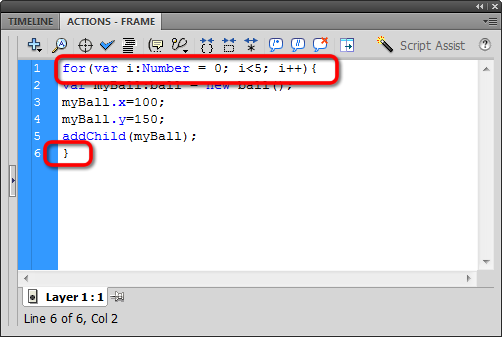# Creating a Loop

## The for loop

We've looked at if statements and else if statements in recent exercises. Now it's time to add another cornerstone to our programming foundation: for loops.

We use the for loop to iterate (or repeat) statements a pre-determined number of times. For loops look like this:

for (init; condition; next){
statements to repeat;
}

The for loop has three expressions: init; condition; next.

Let's start with init (which is short for initialize). We initialize, or start, the for loop by declaring a variable that holds a number. Usually, that variable is i and the number is 0:

var i:Number = 0;

Next comes the condition. You might have an idea of what that is from your understanding of if statements (also called conditional statements). We want the computer to run the statements in our for loop only if the condition is true. This is where we determine how many times we want the for loop to run. If we want the for loop to run five times, we use:

i<5;

Finally, we finish with next, which we use to increment the variable i at the end of every loop.

i++;

We start with i=0. Zero is less than five, satisfying the condition, so an iteration runs. At the end, next adds 1 to i. Now i=1. One is less than five, satisfying the condition, so an iteration runs. At the end, next adds 1 to i, so i = 2. Two is less than five, satisfying the condition, so...

Well, that's why they call it a for LOOP!

Stringing it all together, our for loop likes like this:

for (var i:Number = 0; i<5; i++){
}

Let's use that for loop to repeat our actions five times. Just wrap the curly braces around our existing code. The Actions window should look like this: